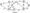# Analysis and Design of Algorithms June 2010

Note: Answer any FIVE full questions, selecting at least TWO questions from each part.

PART-A

1 a. Compare the orders of growth of log2 (n) and Vn . What is your conclusion?

b.  Define O-notation. If fn)  Often)) and f2(n) e 0(g2(n)), prove that f^ + f^tnlGO^axIgnXgsCn)});

c.   Given a positive decimal integer n, write a recursive algorithm which computes the number of binary digits in the binary representation of n. Write the corresponding recurrence relation and solve it.

2 a. Explain the algorithm for selection sort. If A is an array of size n, obtain an expression for the number of key comparisons .

b. Using bubble sort algorithm, arrange the letters of the word ‘QUESTION’ in alphabetical order.

C. Show how divide and conquer technique can be used to compute the product of two n-digit integers. If n is apower of 2, obtain a recurrencerelation for M(n), the number of miihiplicatidns mdsolve it.

3 a. What are the three major variations of decrease arid conquer technique? Explain each with an example.

b.  Sort the letters of the word “EXAMPLE” in alphabetical order using insertion sort.

c.   Describe the Johnson Trotter algorithm for generating permutations. Generate all permutations of (3, 5,7} using the following:

i)  Bottom up minimal change algorithm

ii) Johnson Trotter algorithm.

4 a. Write an algorithm for DFS. With an example, explain how this algorithm can be used to solve topological sorting problem.

b. Using quick sort, arrange the letters of the word “QUICKSORT” in alphabetical order. Show all the steps clearly and draw the tree of the recursive calls made.

PART-B

5 a. Define a 2-3 tree. For any 2-3 tree of height h consisting of n nodes, prove the following: log3 (n +1) -1 < h < log2 (n +1) -1

b.  Describe the algorithm for heap sort.

c.   Show how Horspool’s algorithm can be used to search for the pattern BARBER in a given text. Consider all the four cases.

6 a. Apply Warshall’s algorithm to find the transitive closure of the graph defined by the following adjacency matrix:

b- Using Floyd’s algorithm, solve the all-pairs shortest path problem for the graph whose weight matrix is given below:

C. Using Kruskal’s algorithm, obtain a minimum cost spanning tree for the graph Fig.Q6(c) given below:7 a. Construct a Huffman code for the following data:

 j Character A B D E | Probability 0.4 0.1 0.2 0.15 0.15 Decode the text whose encoding is 10 001011100101

0 using the above Huffman code.

b.   Write short notes on P, NP and NP-complete problems.

c.   Explain how backtracking is used for solving 4 – queens problem. Show the state space tree.

8 a. Solve the following instance of knapsack pro

 Item 1 1 2 3 4 Weight 4 1 7 1 5 3 Value \$40 j \$42 \$25 \$ 12

lem using branch and bound algorithm:

The capacity of the knapsack is W = 10.

b. When does collision occur in hashing? What are the different mechanisms used to resolve collisions?

C What are decision trees? Explain how decision trees are used in sorting algorithms.